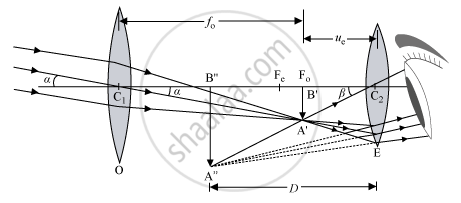Share

# Draw a Labelled Ray Diagram of an Astronomical Telescope to Show the Image Formation of a Distant Object. - Physics

ConceptOptical Instruments Telescope

#### Question

Draw a labelled ray diagram of an astronomical telescope to show the image formation of a distant object. Write the main considerations required in selecting the objective and eyepiece lenses in order to have large magnifying power and high resolution of the telescope.

#### Solution

Astronomical telescope
When the final image is formed at the least distance of distinct vision:Magnifying power, M =β/α

Since α and β are small, we have:

∴ M= tanβ/tanα    ...... (1)

In ΔA'B'C_2, tanβ = (A'B')/(C_2B')

In ΔA'B'C_1, tanβ = (A'B')/(C_2B')

From equation (i), we get:

M = (A'B')/(C_2B') xx (C_1B')/(A'B')

$\Rightarrow$ M = (C_1B')/(C_2B')

Here, C_1B' = +f_0

$\Rightarrow$ C_2B' = -u_e

$\Rightarrow$ M = f_0/ -u_e  .......... (2)

Using the lens equation (1/v-1/u=1/f)for the eyepieces (1/-D-1/-u_e=1/f_e,)we get:

(-1/D+1/u_e=1/f_e)

$\Rightarrow$ (1/u_e=1/(f_e)+1/D)

$\Rightarrow$ (f_0)/u_e =(f_0)/(f_e )(1+f_e/D)

$\Rightarrow$ (-f_0)/u_e =(-f_0)/(f_e )(1+f_e/D) or M = -f_0/(f_e) (1+f_e/D)

In order to have a large magnifying power and high resolution of the telescope, its objective lens should have a large focal length and the eyepiece lens should have a short focal length.

Is there an error in this question or solution?

#### APPEARS IN

Solution Draw a Labelled Ray Diagram of an Astronomical Telescope to Show the Image Formation of a Distant Object. Concept: Optical Instruments - Telescope.
S